Advertisement Remove all ads

# HC Verma solutions for Class 11, 12 Concepts of Physics 1 chapter 6 - Friction [Latest edition]

Advertisement Remove all ads

#### Chapters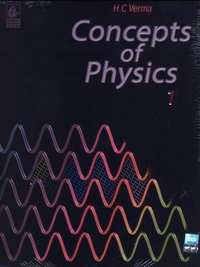## Chapter 6: Friction

Short AnswersMCQExercise
Short Answers [Pages 95 - 96]

### HC Verma solutions for Class 11, 12 Concepts of Physics 1 Chapter 6 Friction Short Answers [Pages 95 - 96]

Short Answers | Q 1 | Page 95

For most of the surfaces used in daily life, the friction coefficient is less than 1. Is it always necessary that the friction coefficient is less than 1?

Short Answers | Q 2 | Page 95

Why is it easier to push a heavy block from behind than to press it on the top and push?

Short Answers | Q 3 | Page 95

What is the average friction force when a person has a usual 1 km walk?

Short Answers | Q 4 | Page 95

Why is it difficult to walk on solid ice?

Short Answers | Q 5 | Page 95

Can you accelerate a car on a frictionless horizontal road by putting more petrol in the engine? Can you stop a car going on a frictionless horizontal road by applying brakes?

Short Answers | Q 6 | Page 95

Spring fitted doors close by themselves when released. You want to keep the door open for a long time, say for an hour. If you put a half kg stone in front of the open door, it does not help. The stone slides with the door and the door gets closed. However, if you sandwitch a 20 g piece of wood in the small gap between the door and the floor, the door stays open. Explain why a much lighter piece of wood is able to keep the door open while the heavy stone fails.

Short Answers | Q 7 | Page 96

A classroom demonstration of Newton's first law is as follows : A glass is covered with a plastic card and a coin is placed on the card. The card is given a quick strike and the coin falls in the glass. (a) Should the friction coefficient between the card and the coin be small or large? (b) Should the coin be light or heavy? (c) Why does the experiment fail if the card is gently pushed?

Short Answers | Q 8 | Page 96

Can a tug of war be ever won on a frictionless surface?

Short Answers | Q 9 | Page 96

Why do tyres have a better grip of the road while going on a level road than while going on an incline?

Short Answers | Q 10 | Page 96

You are standing with your bag in your hands, on the ice in the middle of a pond. The ice is so slippery that it can offer no friction. How can you come out of the ice?

Short Answers | Q 11 | Page 96

When two surfaces are polished, the friction coefficient between them decreases. But the friction coefficient increases and becomes very large if the surfaces are made highly smooth. Explain.

Advertisement Remove all ads
MCQ [Page 96]

### HC Verma solutions for Class 11, 12 Concepts of Physics 1 Chapter 6 Friction MCQ [Page 96]

MCQ | Q 1 | Page 96

In a situation the contact force by a rough horizontal surface on a body placed on it has constant magnitude. If the angle between this force and the vertical is decreased, the frictional force between the surface and the body will

• increase

• decrease

•  remain the same

• may increase or decrease.

MCQ | Q 2 | Page 96

While walking on ice, one should take small steps to avoid slipping. This is because smaller steps ensure

• larger friction

• smaller friction

• larger normal force

• smaller normal force.

MCQ | Q 3 | Page 96

A body of mass M is kept on a rough horizontal surface (friction coefficient = μ). A person is trying to pull the body by applying a horizontal force but the body is not moving. The force by the surface on A is F, where

• F = Mg

• F = μ Mg

• Mg ≤ F ≤ Mga sqrt(1+mu^2)

• Mg ≥ F ≥ Mg sqrt(1-mu^2)

MCQ | Q 4 | Page 96

A scooter starting from rest moves with a constant acceleration for a time ∆t1, then with a constant velocity for the next ∆t2 and finally with a constant deceleration for the next ∆t3 to come to rest. A 500 N man sitting on the scooter behind the driver manages to stay at rest with respect to the scooter without touching any other part. The force exerted by the seat on the man is

• 500 N throughout the journey

• less than 500 N throughout the journey

• more than 500 N throughout the journey

• > 500 N for time ∆t1 and ∆t3 and 500 N for ∆t2.

MCQ | Q 5 | Page 96

Consider the situation shown in figure. The wall smooth but the surface of A and B in contact are rough. The friction on B due to A in equilibrium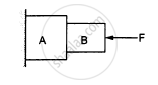• is upward

• is downward

• is zero

• the system cannot remain in equilibrium.

MCQ | Q 6 | Page 96

Suppose all the surface in the previous problem are rough. The direction of friction of B due to A

• is upward

• is downward

• is zero

• depends on the masses of A and B

MCQ | Q 7 | Page 96

Two cars of unequal masses use similar tyres. If they are moving at the same initial speed, the minimum stopping distance

• is smaller for the heavier car

• is smaller for the lighter car

• is same for both cars

• depends on the volume of the car

MCQ | Q 8 | Page 96

In order to stop a car in shortest distance on a horizontal road, one should

• apply the brakes very hard so that the wheels stop rotating

• apply the brakes hard enough to just prevent slipping

• pump the brakes (press and release)

• shut the engine off and not apply brakes

MCQ | Q 9 | Page 96

A block A kept on an inclined surface just begins to slide if the inclination is 30°. The block is replaced by another block B and it is found that it just begins to slide if the inclination is 40°.

• mass of A > mass of B

• mass of A < mass of B

• mass of A = mass of B

• all of three are possible.

MCQ | Q 10 | Page 96

A boy of mass M is applying a horizontal force to slide a box of mass M' on a rough horizontal surface. The coefficient of friction between the shoes of the boy and the floor is μ and that between the box and the floor is μ'. In which of the following cases it is certainly not possible to slide the box?

• μ < μ', M < M'

• μ > μ', M < M'

• μ < μ', M > M'

• μ > μ', M > M'

Advertisement Remove all ads
MCQ [Page 97]

### HC Verma solutions for Class 11, 12 Concepts of Physics 1 Chapter 6 Friction MCQ [Page 97]

MCQ | Q 1 | Page 97

Let F, FN and f denote the magnitudes of the contact force, normal force and the friction exerted by one surface on the other kept in contact. If none of these is zero.
(a) F > FN
(b) F > f
(c) FN > f
(d) FN − f < F < FN + f.

MCQ | Q 2 | Page 97

The contact force exerted by a body A on another body B is equal to the normal force between the bodies We conclude that
(a) the surface must be frictionless
(b) the force of friction between the bodies is zero
(c) the magnitude of normal force equal that of friction
(d) the bodies may be rough but they don't slip on each other.

MCQ | Q 3 | Page 97

Mark the correct statements about the friction between two bodies.
(a) Static friction is always greater than the kinetic friction.
(b) Coefficient of static friction is always greater than the coefficient of kinetic friction.
(c) Limiting friction is always greater than the kinetic friction.
(d) Limiting friction is never less than static friction.

MCQ | Q 4 | Page 97

A block is placed on a rough floor and a horizontal force F is applied on it. The force of friction f by the floor on the block is measured for different values of F and a graph is plotted between them.
(a) The graph is a straight line of slope 45°.
(b) The graph is a straight line parallel to the F-axis.
(c) The graph is a straight line of slope 45° for small F and a straight line parallel to the F-axis for large F.
(d) There is a small kink on the graph.

MCQ | Q 5 | Page 97

Consider a vehicle going on a horizontal road towards east. Neglect any force by the air. The frictional force on the vehicle by the road
(a) is towards east if the vehicle is accelerating
(b) is zero if the vehicle is moving with a uniform velocity
(c) must be towards east
(d) must be towards west.

Advertisement Remove all ads
Exercise [Pages 97 - 99]

### HC Verma solutions for Class 11, 12 Concepts of Physics 1 Chapter 6 Friction Exercise [Pages 97 - 99]

Exercise | Q 1 | Page 97

A body slipping on a rough horizontal plane moves with a deceleration of 4.0 m/s2. What is the coefficient of kinetic friction between the block and the plane?

Exercise | Q 2 | Page 97

A block is projected along a rough horizontal road with a speed of 10 m/s. If the coefficient of kinetic friction is 0.10, how far will it travel before coming to rest?

Exercise | Q 3 | Page 97

A block of mass m is kept on a horizontal table. If the static friction coefficient is μ, find the frictional force acting on the block.

Exercise | Q 4 | Page 97

A block slides down an inclined surface of inclination 30° with the horizontal. Starting from rest it covers 8 m in the first two seconds. Find the coefficient of kinetic friction between the two.

Exercise | Q 5 | Page 97

Suppose the block of the previous problem is pushed down the incline with a force of 4 N. How far will the block move in the first two seconds after starting from rest? The mass of the block is 4 kg.

Exercise | Q 6 | Page 97

A body of mass 2 kg is lying on a rough inclined plane of inclination 30°. Find the magnitude of the force parallel to the incline needed to make the block move (a) up the incline (b) down the incline. Coefficient of static friction = 0.2.

Exercise | Q 7 | Page 97

Repeat part (a) of problem 6 if the push is applied horizontally and not parallel to the incline.

Exercise | Q 8 | Page 97

In a children-park an inclined plane is constructed with an angle of incline 45° in the middle part (in the following figure). Find the acceleration of boy sliding on it if the friction coefficient between the cloth of the boy and the incline is 0.6 and g = 19 m/s2.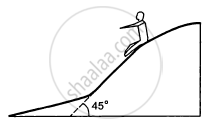Exercise | Q 9 | Page 97

A body starts slipping down an incline and moves half metre in half second. How long will it take to move the next half metre?

Exercise | Q 10 | Page 97

The angle between the resultant contact force and the normal force exerted by a body on the other is called the angle of friction. Show that, if λ be the angle of friction and μ the coefficient of static friction λ ≤ tan−1 μ.

Exercise | Q 11 | Page 97

Consider the situation shown in the following figure. Calculate (a) the acceleration of the 1.0 kg blocks, (b) the tension in the string connecting the 1.0 kg blocks and (c) the tension in the string attached to 0.50 kg.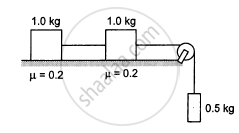Exercise | Q 12 | Page 98

If the tension in the string in the following figure is 16 N and the acceleration of each block is 0.5 m/s2, find the friction coefficients at the two contact with the blocks.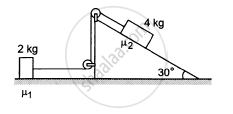Exercise | Q 13 | Page 98

The friction co-efficient between the table and the block shown in the following figure is 0.2. Find the tensions in the two strings.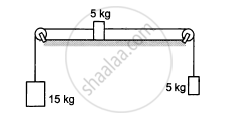Exercise | Q 14 | Page 98

The friction coefficient between a road and the type of a vehicle is 4/3. Find the maximum incline the road may have so that once had brakes are applied and the wheel starts skidding, the vehicle going down at a speed of 36 km/hr is stopped within 5 m.

Exercise | Q 15 | Page 98

The friction coefficient between an athelete's shoes and the ground is 0.90. Suppose a superman wears these shoes and races for 50 m. There is no upper limit on his capacity of running at high speeds. (a) Find the minimum time that he will have to take in completing the 50 m starting from rest. (b) Suppose he takes exactly this minimum time to complete the 50 m, what minimum time will he take to stop?

Exercise | Q 16 | Page 98

A care is going at a speed of 21.6 km/hr when it encounters at 12.8 m long slope of angle 30° (in the following figure). The friction coefficient between the road and the tyre is 1/2sqrt3. Show that no matter how hard the driver applies the brakes, the car will reach the bottom with a speed greater than 36 km/hr. Take g = 10 m/s2.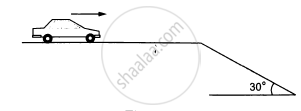Exercise | Q 17 | Page 98

A car starts from rest on a half kilometre long bridge. The coefficient of friction between the tyre and the road is 1.0. Show that one cannot drive through the bridge in less than 10 s.

Exercise | Q 18 | Page 98

In the following figure shows two blocks in contact sliding down an inclined surface of inclination 30°. The friction coefficient between the block of mass 2.0 kg and the incline is μ1, and that between the block of mass 4.0 kg and incline is μ2. Calculate the acceleration of the 2.0 kg block if (a) μ1 = 0.20 and μ2 = 0.30, (b) μ1 = 0.30 and μ2 = 0.20. Take g = 10 m/s2.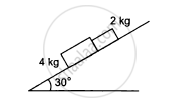Exercise | Q 19 | Page 98

Two masses M1 and M2 are connected by a light rod and the system is slipping down a rough incline of angle θ with the horizontal. The friction coefficient at both the contacts is μ. Find the acceleration of the system and the force by the rod on one of the blocks.

Exercise | Q 20 | Page 98

A block of mass M is kept on a rough horizontal surface. The coefficient of static friction between the block and the surface is μ. The block is to be pulled by applying a force to it. What minimum force is needed to slide the block? In which direction should this force act?

Exercise | Q 21 | Page 98

The friction coefficient between the board and the floor shown in the following figure is μ. Find the maximum force that the man can exert on the rope so that the board does not slip on the floor.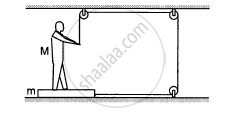Exercise | Q 22 | Page 98

A 2 kg block is placed over a 4 kg block and both are placed on a smooth horizontal surface. The coefficient of friction between the block is 0.20. Find the acceleration of the two blocks if a horizontal force of 12 N is applied to (a) the upper block, (b) the lower block. Take g = 10 m/s2.

Exercise | Q 23 | Page 98

Find the accelerations a1, a2, a3 of the three blocks shown in the following figure if a horizontal force of 10 N is applied on (a) 2 kg block, (b) 3 kg block, (c) 7 kg block. Take g = 10 m/s2.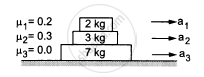Exercise | Q 24 | Page 98

The friction coefficient between the two blocks shown in the following figure is μ but the floor is smooth. (a) What maximum horizontal force F can be applied without disturbing the equilibrium of the system? (b) Suppose the horizontal force applied is double of that found in part (a). Find the accelerations of the two masses.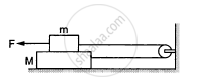Exercise | Q 25 | Page 99

Suppose the entire system of the previous questions is kept inside an elevator which is coming down with an acceleration a < g. Repeat parts (a) and (b).

Exercise | Q 26 | Page 99

Consider the situation shown in the following figure. Suppose a small electric field E exists in the space in the vertically charge Q on its top surface. The friction coefficient between the two blocks is μ but the floor is smooth. What maximum horizontal force F can be applied without disturbing the equilibrium?
[Hint: The force on a charge Q bye the electric field E is F = QE in the direction of E.]Exercise | Q 27 | Page 99

A block of mass m slips on a rough horizontal table under the action of a horizontal force applied to it. The coefficient of friction between the block and the table is μ. The table does not move on the floor. Find the total frictional force applied by the floor on the legs of the table. Do you need the friction coefficient between the table and the floor or the mass of the table?

Exercise | Q 28 | Page 99

Find the acceleration of the block of mass M in the situation of figure in the following. The coefficient of friction between the two blocks is μ1 and that between the bigger block and the ground is μ2.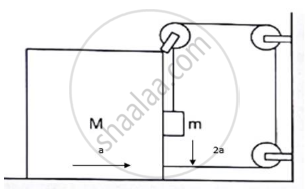Exercise | Q 29 | Page 99

A block of mass 2 kg is pushed against a rough vertical wall with a force of 40 N, coefficient of static friction being 0.5. Another horizontal force of 15 N, is applied on the block in a direction parallel to the wall. Will the block move? If yes, in which direction? If no, find the frictional force exerted by the wall on the block.

Exercise | Q 30 | Page 99

A person (40 kg) is managing to be at rest between two vertical walls by pressing one wall A by his hands and feet and the other wall B by his back (in the following figure). Assume that the friction coefficient between his body and the walls is 0.8 and that limiting friction acts at all the contacts. (a) Show that the person pushes the two wall with equal force. (b) Find the normal force exerted by either wall on the person. Take g = 10 m/s2.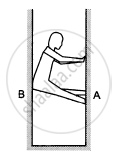Exercise | Q 31 | Page 99

In the following figure shows a small block of mass m kept at the left end of a larger block of mass M and length l. The system can slide on a horizontal road. The system is started towards right with an initial velocity v. The friction coefficient between the road and the bigger block is μ and that between the block is μ/2. Find the time elapsed before the smaller blocks separates from the bigger block.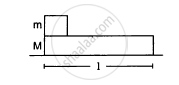Advertisement Remove all ads

## Chapter 6: Friction

Short AnswersMCQExercise## HC Verma solutions for Class 11, 12 Concepts of Physics 1 chapter 6 - Friction

HC Verma solutions for Class 11, 12 Concepts of Physics 1 chapter 6 (Friction) include all questions with solution and detail explanation. This will clear students doubts about any question and improve application skills while preparing for board exams. The detailed, step-by-step solutions will help you understand the concepts better and clear your confusions, if any. Shaalaa.com has the CBSE Class 11, 12 Concepts of Physics 1 solutions in a manner that help students grasp basic concepts better and faster.

Further, we at Shaalaa.com provide such solutions so that students can prepare for written exams. HC Verma textbook solutions can be a core help for self-study and acts as a perfect self-help guidance for students.

Concepts covered in Class 11, 12 Concepts of Physics 1 chapter 6 Friction are Lubrication - (Laws of Motion), Law of Conservation of Linear Momentum and Its Applications, Aristotle’s Fallacy, The Law of Inertia, Newton'S First Law of Motion, Newton’s Second Law of Motion, Newton's Third Law of Motion, Conservation of Momentum, Equilibrium of a Particle, Common Forces in Mechanics, Circular Motion, Solving Problems in Mechanics, Static and Kinetic Friction, Laws of Friction, Inertia, Intuitive Concept of Force, Dynamics of Uniform Circular Motion - Centripetal Force, Examples of Circular Motion (Vehicle on a Level Circular Road, Vehicle on a Banked Road), Rolling Friction, Introduction.

Using HC Verma Class 12 solutions Friction exercise by students are an easy way to prepare for the exams, as they involve solutions arranged chapter-wise also page wise. The questions involved in HC Verma Solutions are important questions that can be asked in the final exam. Maximum students of CBSE Class 12 prefer HC Verma Textbook Solutions to score more in exam.

Get the free view of chapter 6 Friction Class 12 extra questions for Class 11, 12 Concepts of Physics 1 and can use Shaalaa.com to keep it handy for your exam preparation

Advertisement Remove all ads
Share
Notifications

View all notifications

Forgot password?